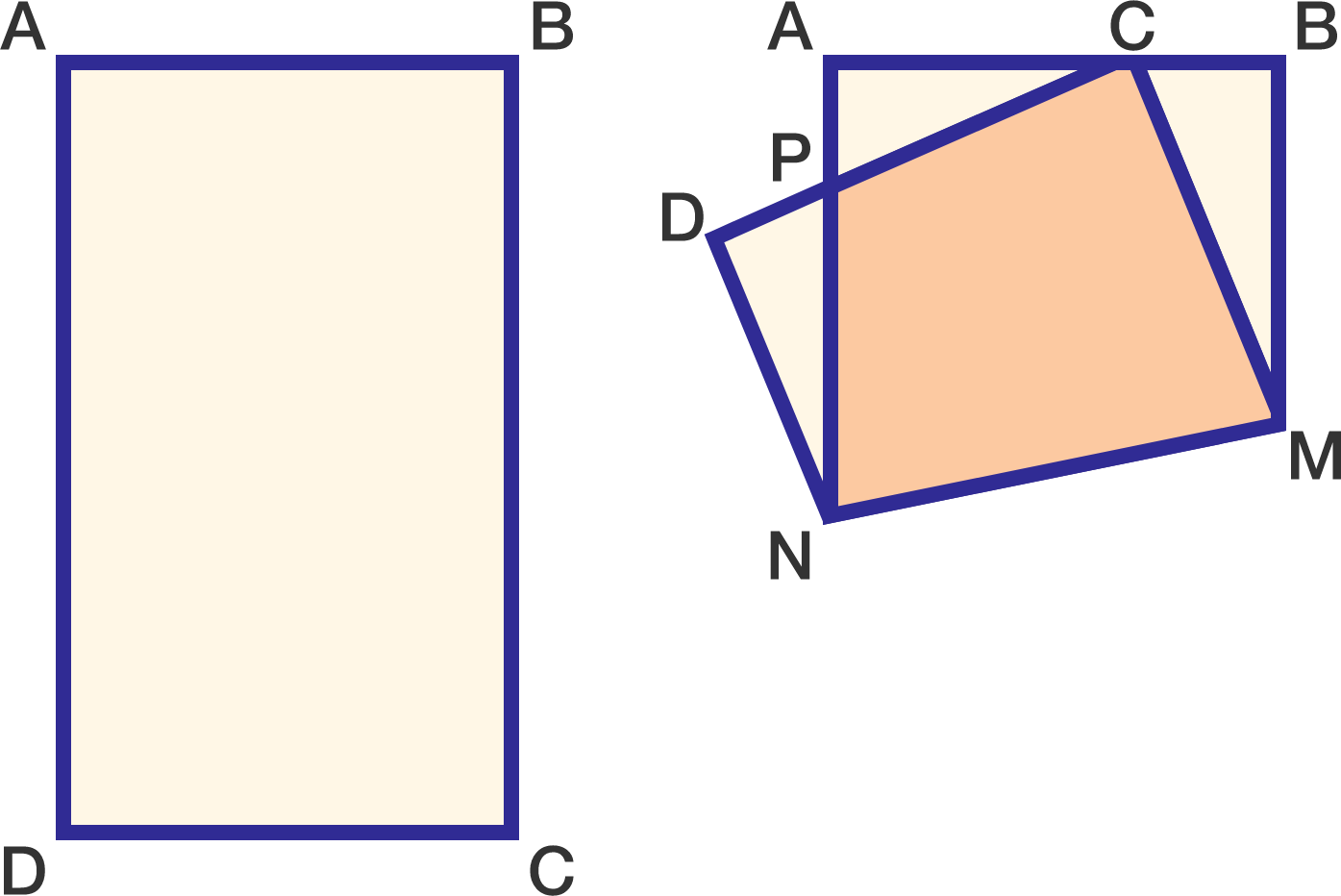In rectangle ABCD (below left), $AB=3$ and $BC=5$. The corner at C is folded up (below right) and lands on $\overline{AB}$ so that $AC=2$ and $CB=1$.The area of quadrilateral CPNM can be written as $\frac{m}{n}$, where m and n are positive, coprime integers. Find $m+n$.

×